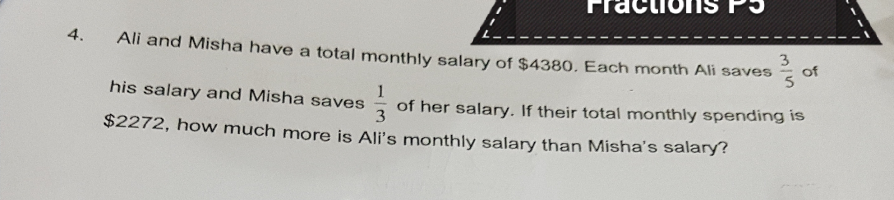# QuestionTq😁

This is a Simultaneous equation.

 Spend R1 Total R2 R1 × 3 R2 × 2 R2 – R1 Ali 2 5 6 10 4 Misha 2 3 6 6 0 Amount 2272 4380 6816 8760 1944

4u = 1944

1u = 1944 ÷ 4 = 486

5u = 486 × 5 = 2430 (Ali)

4380 – 2430 = 1950 (Misha)

2430 – 1950 = 480

0 Replies 0 Likes ✔Accepted Answer